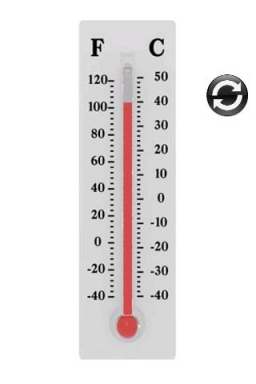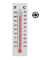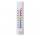# Change in temperature

Starting temperature is 21°C, the highest temperature is 32°C. What is the change in temperature?

d =  11 °C

### Step-by-step explanation:Did you find an error or inaccuracy? Feel free to write us. Thank you!

Showing 1 comment:Math student
If the temp is 12 degrees Fahrenheit and changes by -26 degrees Fahrenheit, what is the temp now?## Related math problems and questions:

• The bathDad poured 50 liters of water at 60°C into the tub. How much cold water must be added at 10°C to obtain a bath that has a temperature of 40°C?
• Temperature change 3At 2 PM, The temperature was 76 degrees Fahrenheit. At 8 PM, the temperature was 58 degrees Fahrenheit. What was the change in temperature?
• Mixing Celsius and FahrenheitAdd up three temperatures: 5°F +6°F +0°C
• Now isNow is 5°C morning. What will the temperature be if i. It rises by 12°C ii. It falls by 15°C
• The temperature 3The temperature is -12 degrees Fahrenheit. During the following 5 hours, the temperature decrease by 23 degrees Fahrenheit. What is the weather at 11 pm?
• OpheliaOphelia recorded the temperature of a cold store every two hours. 1. At 6 am, it was -4°C, and at 8 am, it was -1°C. By how much did the temperature rise? 2. The temperature went up by 5°C in the next two hours. .What was the temperature at 10 am?In about 12 hours in North Dakota the temperature rose from -33 degrees farenheit to 50 degrees farenheit. By how much did the temperature change?
• Outside temperatureThe temperature outside was 57 degree Fahrenheit. During the next few hours it decreased by 18 degrees and then increased by 23 degrees. Find new temperature.
• Celsius 25 degrees Celsius at midday dropped 12°Celsius degree by evening. What is the temperature?
• Temperature up and dowmAt 6 AM, the temperature is 19.4°C. Between 6 AM and noon, the temperature rises 3.8°C. Between noon and 6 PM, the temperature falls 2.5°C. What is the temperature at 6 PM?
• Temperature variationsToday's temperature was 80 degrees, and then the temperature dropped 10 degrees. Then it dropped 15 degrees again, then the next day, the temperature went up 2 degrees. What would the temperature be?
• Temperature rise and fallOn Friday, the temperature was 82°F. The temperature changed by –2°F on Saturday, and then it changed by 5°F on Sunday. What was the temperature on Sunday? How did the temperature change? Note we consider the mathematical problem formulated in this way to
• ThermometerThe thermometer showed -12 degrees Celsius in the morning then the temperature rises by 4 degrees and later again increased by 2 degrees at the evening has fallen by 5 degrees and then falls 3 degrees. What end temperature does the thermometer show?
• The temperature 6The temperature was 47°F on Thursday and 60°F on Friday. How much did the temperature rise?
• FreezerThe temperature is -8 degrees Celsius in the freezer. The outside temperature is 2 degrees Celsius. One minute took to change the temperature by one degree Celsius if we open the door. How many minutes the temperature in the freezer will rise?
• At midmorningThe temperature at midmorning was 31°C. Early in the evening, the temperature dropped by 5°C. What was the resulting temperature?
• NoontimeIn the morning temperature was 110°F. By noontime, it has gone up by 15°F. What was the noon temperature? (Give your answer in °F)